# Manual

## Introduction

The package varycoef contains methods to model and estimate varying coefficients. In its current version 0.3.0 it supports:

• only spatially varying coefficient (SVC)

• different MLE approaches to model SVC and to give predictions.

The methodology is based on (Dambon, Sigrist, and Furrer 2021).

### Spatially Varying Coefficient Models

We define a full SVC model as

$y(s) = x^{(1)}(s)\beta_1(s) + ... + x^{(p)}(s)\beta_p(s) + \epsilon(s)$

with the coefficients represented by Gaussian random fields (GRF) $$\beta_j(\cdot) \sim \mathcal N (\mu_j \textbf 1_n, C_j(\cdot, \cdot))$$. That is, every coefficient $$j = 1, ..., p$$ is distinctly defined by a mean $$\mu_j$$ and a covariance matrix defined by an underlying covariance function $$C_j(s_k, s_l) = \sigma_j^2 \phi_{\rho_j}(s_k, s_l)$$, where $$\sigma_j^2$$ is the variance and $$\rho_j$$ is the scale of the GRF. Further, $$\epsilon$$ is a nugget effect with variance $$\tau^2$$.

However, there are some cases, where the assumption of a full SVC model is not applicable. We want to give options for covariates w.r.t.:

1. mixed SVC, i.e. as above with its respective mean (fixed effect) and random effect described by its GRF.
2. fixed effect, i.e. without an GRF modelling its spatial structure.
3. mean-zero SVC, i.e. only a zero-mean random effect modelling the spatial structure.

That is why we generalize the model above. First, note that we can write it in matrix form as

$\textbf y(\textbf s) = \textbf X \beta(\textbf s) + \epsilon( \textbf s)$

where $$\textbf X$$ is our model matrix. Then we can write down the model divided into an fixed effect part and a random effect part:

$\textbf y(\textbf s) = \textbf X \mu + \textbf W \eta(\textbf s) + \epsilon( \textbf s)$

where $$\eta$$ is the joint mean-zero GRF. Note that both model are the same if $$\textbf X = \textbf W$$. Thus, we can specify options 1 to 3 from above by in- or excluding columns of $$\textbf X$$ or $$\textbf W$$, respectively.

### Example

To give a simple example, we start by sampling artificial data. So define an full SVC model as given above and sample from a regular grid using a help function:

# number of SVC
p <- 3

(pars <- data.frame(mu = rep(0, p),
var = c(0.1, 0.2, 0.3),
scale = c(0.3, 0.1, 0.2)))
##   mu var scale
## 1  0 0.1   0.3
## 2  0 0.2   0.1
## 3  0 0.3   0.2
nugget.var <- 0.05

# sqrt of total number of observations
m <- 20

# function to sample SVCs
sp.SVC <- fullSVC_reggrid(m = m, p = p,
cov_pars = pars,
nugget = nugget.var)

library(sp)
# visualization
spplot(sp.SVC, colorkey = TRUE)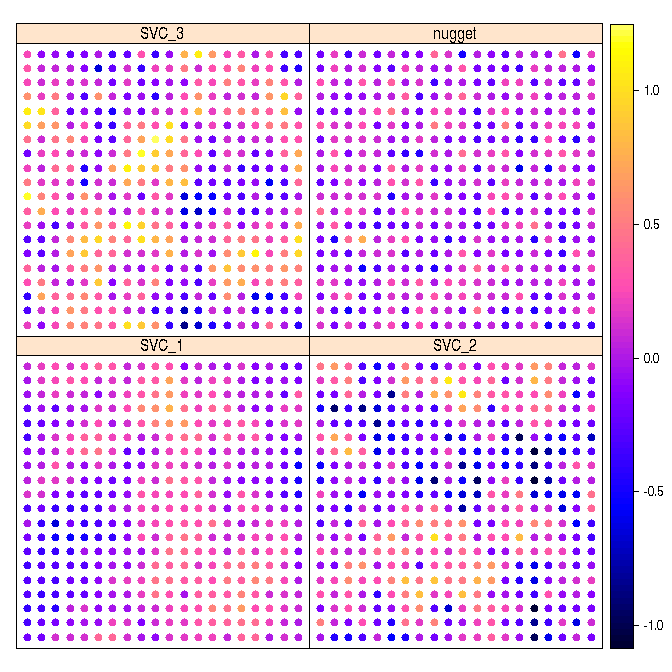We further need some covariates which we sample from a standard normal. In order to model an intercept, we set $$x^{(1)} = 1$$:

# total number of observation
n <- m^2

X <- matrix(c(rep(1, n), rnorm((p-1)*n)), ncol = p)
head(X)
##      [,1]       [,2]       [,3]
## [1,]    1 -0.2890233 -0.5116037
## [2,]    1  0.6565134  0.2369379
## [3,]    1 -0.4539977 -0.5415892
## [4,]    1 -0.5938646  1.2192276
## [5,]    1 -1.7103797  0.1741359
## [6,]    1 -0.2094484 -0.6152683

We compute the response $$y$$:

y <- apply(X * as.matrix(sp.SVC@data[, 1:p]), 1, sum) + sp.SVC@data[, p+1]

## MLE in varycoef

The main function of this package is SVC_mle. Its function call starts the MLE but it requires some preparation and settings of control parameters. We go through each argument of the SVC_mle function and its control parameter SVC_mle.control.

### The Function SVC_mle

As one might see in the help file of the SVC_mle function, it has 3 mandatory arguments: y, the response; X, the data matrix and locs, the locations. If we do not change W, i.e. W = NULL, then we use W = X and are in the case of a full SVC. We will give examples for different kinds of models.

### Control Parameters

As for the control parameters for SVC_mle, we go through them as they are implemented in the current version of varycoef. By calling SVC_mle_control, we create an list with all needed arguments to start a simple SVC_mle.

control <- SVC_mle_control()
str(control)
## List of 13
##  $cov.name : chr "exp" ##$ tapering   : NULL
##  $parallel : NULL ##$ init       : NULL
##  $lower : NULL ##$ upper      : NULL
##  $save.fitted: logi TRUE ##$ profileLik : logi FALSE
##  $mean.est : chr "GLS" ##$ pc.prior   : NULL
##  $extract_fun: logi FALSE ##$ hessian    : logi FALSE
##  $dist :List of 1 ## ..$ method: chr "euclidean"

#### Covariance Function

Here we define the covariance function $$C_j(s_k, s_l) = \sigma_j^2 \phi_{\rho_j}(s_k, s_l)$$. In its current version 0.3.0, varycoef supports only exponential covariance functions, i.e. $$\phi_{\rho}(s_k, s_l) = \exp\left(\frac{\|s_k - s_l\|}{\rho}\right)$$.

#### Tapering

Covariance tapering goes back to (Furrer, Genton, and Nychka 2006) and is a technique to challenge the “big $$n$$ problem” when dealing with spatial data. When working with $$n$$ observations, the covariance matrix has dimension $$n \times n$$. The likelihood function of a single GRF or, in particular, the SVC models as described above, includes the inverse as well as the determinant of a covariance matrix. The computation of both is based on the Cholesky decomposition which has run-time $$\mathcal O(n^3)$$. There are several ways on how to deal with this computational burden.

With covariance tapering, we introduce a sparsity structure on the covariance matrix. This sparsity structure can then be used to our advantage with the Cholesky decomposition implemented in the package spam, (Furrer and Sain 2010). In a nutshell, this decomposition becomes faster as the sparsity increases. However, this comes with a trade-off in the precision of the MLE of the covariance parameters with finitely many observation. Asymptotically, the procedure is consistent.

By default, the tapering entry is NULL, i.e. no tapering is applied. If a scalar is provided, then this is taper range is applies. In other words, every spatial dependency is cut for distances larger than tapering. We illustrate the difference between both the untapered and tapered covariance matrix of the SVC on the regular grid example from above. The function fullSVC_reggrid is used to sample SVCs for a full SVC model.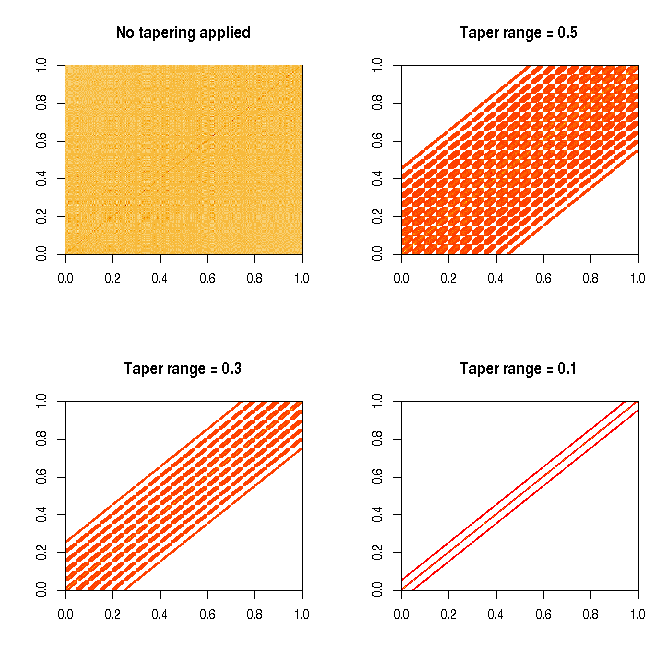Finally, we show the time differences of evaluating the likelihood function between the different taper ranges. We use the microbenchmark package:

library(microbenchmark)

(mb <- microbenchmark(no_tapering  = out[][](),
tapering_0.5 = out[][](),
tapering_0.3 = out[][](),
tapering_0.1 = out[][]())
)
## Unit: milliseconds
##          expr      min       lq     mean   median       uq      max neval cld
##   no_tapering 49.58213 50.37040 62.00703 53.39572 54.61746 240.6759   100   b
##  tapering_0.5 53.36417 56.38514 72.74536 57.52913 59.23605 257.9709   100   b
##  tapering_0.3 32.73997 36.53168 43.90478 37.28464 40.21388 226.9037   100  a
##  tapering_0.1 20.93598 24.67909 29.89846 25.29569 28.97936 210.6243   100  a
boxplot(mb, unit = "ms", log = TRUE, xlab = "tapering", ylab = "time (milliseconds)")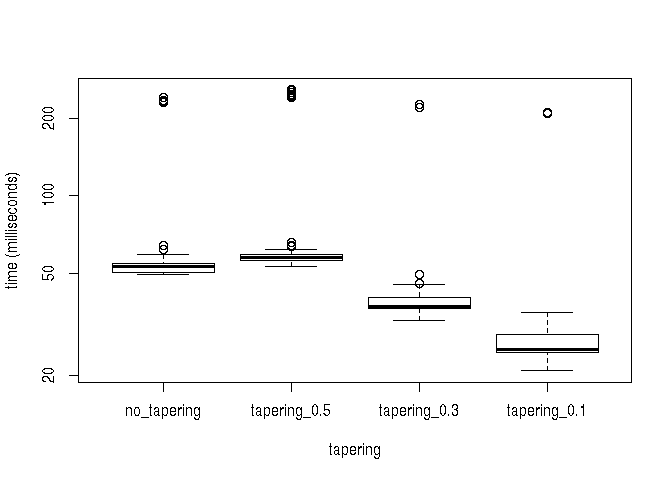#### Initial Values

To run an MLE, we need initial values for all variances and the scales as well as the means. A good starting point for the means are the estimated coefficients of an OLS, i.e.:

(mu.init <- coef(lm(y~.-1, data = data.frame(y = y, X = X))))
##        X.1        X.2        X.3
## 0.04638084 0.02147242 0.17528015

For the variance, we take the estimated variance of the OLS method, i.e

(var.init <- sigma(lm(y~.-1, data = data.frame(y = y, X = X)))^2)
##  0.3999115

For the scale, we remind ourselves that the effective range of an exponential GRF is approximately 3 times its range. Since in our case we are in an restricted domain of the unit square, any dependency structure on a distance above the maximum distance in the domain, we cannot model. Therefore we suggest to take an initial scale of

scale.init <- 0.2

The vector of initial values is then:

init <- c(
# GRFs scales and variances
rep(c(scale.init, var.init), p),
# nugget variance
var.init,
# means
mu.init)

One can either overwrite the default initial value (control$init) or call SVC_mle_control with corresponding arguments: # default control$init
## NULL
# overwrite
control$init <- init # create new control <- SVC_mle_control(init = init) The default values gives initial values of $$0.3$$ for the variances and ranges and $$0$$ for the means, which might not be the best option in general. #### Lower and Upper Bounds The optimization function optim allows with its chosen method "L-BFGS-B" the usage of lower and upper bounds. The lower and upper bounds for each parameter can be given in correspondence to the order in the initial values. #### Save Fitted Values The argument save.fitted takes logical values and decides whether the fitted values of the SVC model after the MLE should be computed. This takes some extra time, but can be useful since an extraction of those fitted values as well as the residuals is possible using a methods fitted or residuals. In case of allocation errors, set to FALSE. # default control$save.fitted
##  TRUE
# overwrite
control$save.fitted <- TRUE #### Profile Likelihood In some SVC models, it might be an advantage to optimize not over all parameters, i.e. the mean and the covariance parameters. The motivation here is a dimension reduction of the parameter space. Setting profileLik to TRUE, the optimization will be done using the profile likelihood of the covariance parameters. The mean effects are then implicitly calculated using the generalized least squares estimate. # default control$profileLik
##  FALSE

Attention: Since the number of parameters is reduced, one should also be aware that the initial values as well as the lower and upper bounds are given correctly.

#### Regularizing Priors

We implemented a usage of penalizing complexity priors. The argument pc.prior takes a vector of length 4 as an input where the values are $$\rho_0, \alpha_\rho, \sigma_0, \alpha_\sigma$$ to compute penalized complexity priors. One wants to penalize the lower tail on the range parameter as well as the upper tail of the standard deviation:

$P(\rho < \rho_0) = \alpha_\rho, \quad P(\sigma > \sigma_0) = \alpha_\sigma.$

#### Parallelization

With version 0.2.10 varycoef is now able to parallelize the likelihood optimization. In each iteration step the objective function, i.e., a modified likelihood, has to be evaluated at several points in a small neighborhood. Using the package optimParallel (Gerber and Furrer 2019), is can be done simultaneously. The procedure to do so is the following:

1. Initialize a cluster by parallel::makeCluster.

2. Create list containing this cluster, as one would with optimParallel::optimParallel. In this list other arguments towards the function optimParallel can be passed, see help file.

3. Set argument parallel to the created list.

4. Run SVC_mle as usual.

5. Make sure to stop cluster afterwards.

The code looks something like that:

require(varycoef)
require(parallel)
require(optimParallel)

# step 1: initialize cluster
cl <- makeCluster(detectCores()-1)

# step 2: create optimParallel control
parallel.control <- list(cl = cl, forward = TRUE, loginfo = FALSE)

# step 3: add control containing optimParallel controls
control.p <- control
control.p$parallel <- parallel.control # step 4: run SVC_mle fit.p <- SVC_mle(y = y, X = X, locs = coordinates(sp.SVC), control = control.p) # step 5: stop cluster stopCluster(cl); rm(cl) summary(fit.p) rm(control.p, fit.p) #### Extract Function In some situations, it is useful to extract the objective function before starting the optimization itself. For instance, (Gerber and Furrer 2019) states that the overhead of the parallelization set up results in a faster optimization only if the evaluation time of a single objective function is greater than 0.05 seconds. Another example where the extracted function is needed are machine specific issues regarding the optimization. ### Example: MLE of full SVC We can now start the MLE . The following function call takes a few second. fit <- SVC_mle(y = y, X = X, locs = coordinates(sp.SVC), control = control) The received object fit is of class SVC_mle. For this class, there are numerous methods such as: # estimated ... # ... covariance parameters cov_par(fit) ## SVC1.range SVC1.var SVC2.range SVC2.var SVC3.range SVC3.var nugget.var ## 0.12231550 0.04895641 0.07934721 0.15376355 0.07486083 0.18421108 0.03180938 ## attr(,"GRF") ##  "exp"  # ... mean effects coef(fit) ## Var1 Var2 Var3 ## 0.04744684 -0.01592360 0.18216363 # summary summary(fit) ## ## Call: ## SVC_mle with 3 fixed effect(s) and 3 SVC(s) ## using 400 observations at 400 different locations. ## ## Residuals: ## Min. 1st Qu. Median 3rd Qu. Max. ## -0.19545 -0.04729 -0.00242 0.04794 0.25614 ## ## Residual standard error (nugget effect): 0.1784 ## Multiple R-squared: 0.9861 ## ## ## Coefficients of fixed effects: ## Var1 Var2 Var3 ## 0.04745 -0.01592 0.18216 ## ## ## Covariance parameters of the SVC(s): ## range variance ## SVC1 0.12232 0.04896 ## SVC2 0.07935 0.15376 ## SVC3 0.07486 0.18421 ## ## The covariance parameters were estimated for the GRFs using ## exp. covariance functions. No covariance tapering applied. ## ## ## MLE: ## The MLE terminated after 87 function evaluations with convergence code 0 ## (0 meaning that the optimization was succesful). ## The final likelihood value for 6 parameters is -290.3. # residual plots oldpar <- par(mfrow = c(1, 2)) plot(fit, which = 1:2)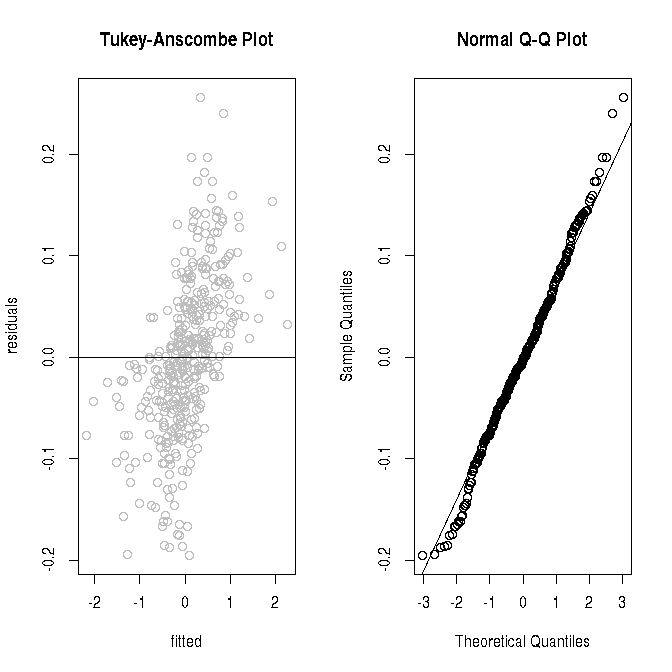par(mfrow = c(1, 1)) plot(fit, which = 3)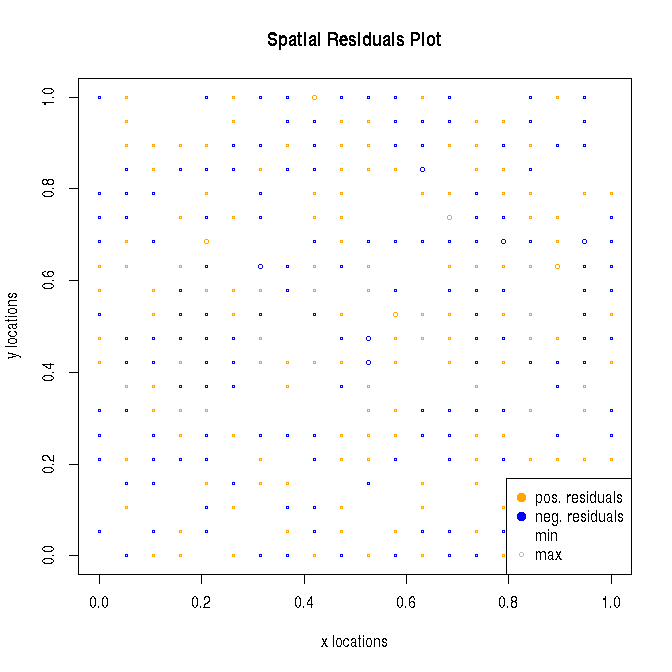par(oldpar) Now, we can use our fit object to make predictions: # calling predictions without specifying new locations (newlocs) or # new covariates (newX) gives estimates of SVC only at the training locations. pred.SVC <- predict(fit) Since we know the true SVC, we can compute the error in prediction and compare it to the true values. colnames(pred.SVC)[1:p] <- paste0("pred.",colnames(pred.SVC)[1:p]) coordinates(pred.SVC) <- ~loc_x+loc_y all.SVC <- cbind(pred.SVC, sp.SVC[, 1:3]) # compute errors all.SVC$err.SVC_1 <- all.SVC$pred.SVC_1 - all.SVC$SVC_1
all.SVC$err.SVC_2 <- all.SVC$pred.SVC_2 - all.SVC$SVC_2 all.SVC$err.SVC_3 <- all.SVC$pred.SVC_3 - all.SVC$SVC_3

colnames(all.SVC@data) <- paste0(rep(c("pred.", "true.", "err."), each = p), "SVC_", rep(1:p, 3))

spplot(all.SVC[, paste0(rep(c("true.", "err.", "pred."), each = p),
"SVC_", 1:p)], colorkey = TRUE)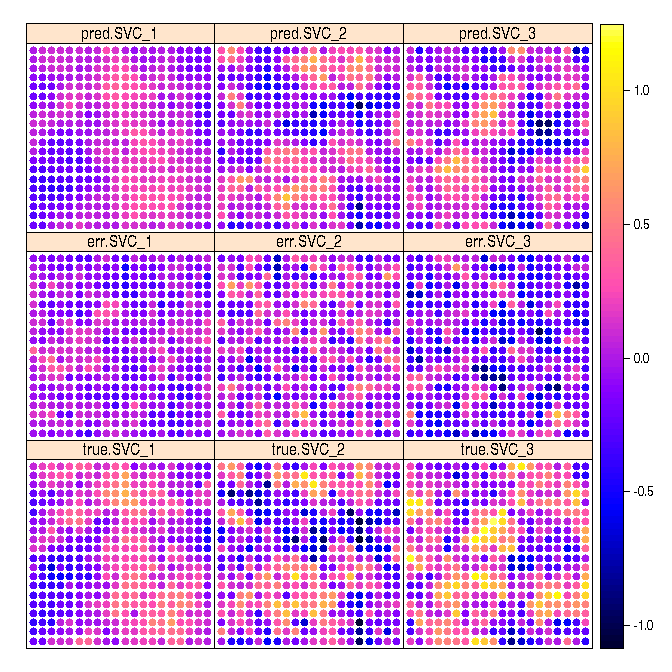In this small example we already can see that the predicted SVC takes the general spatial structure of the true SVC. The error does not appear to have spatial structure for the SVC 2 and 3, respectively. However, the error for the intercept seems to have some spatial structure. If we increase the number of observations, the picture changes:

knitr::include_graphics("figures/SVCs_result_n2500_p3.png")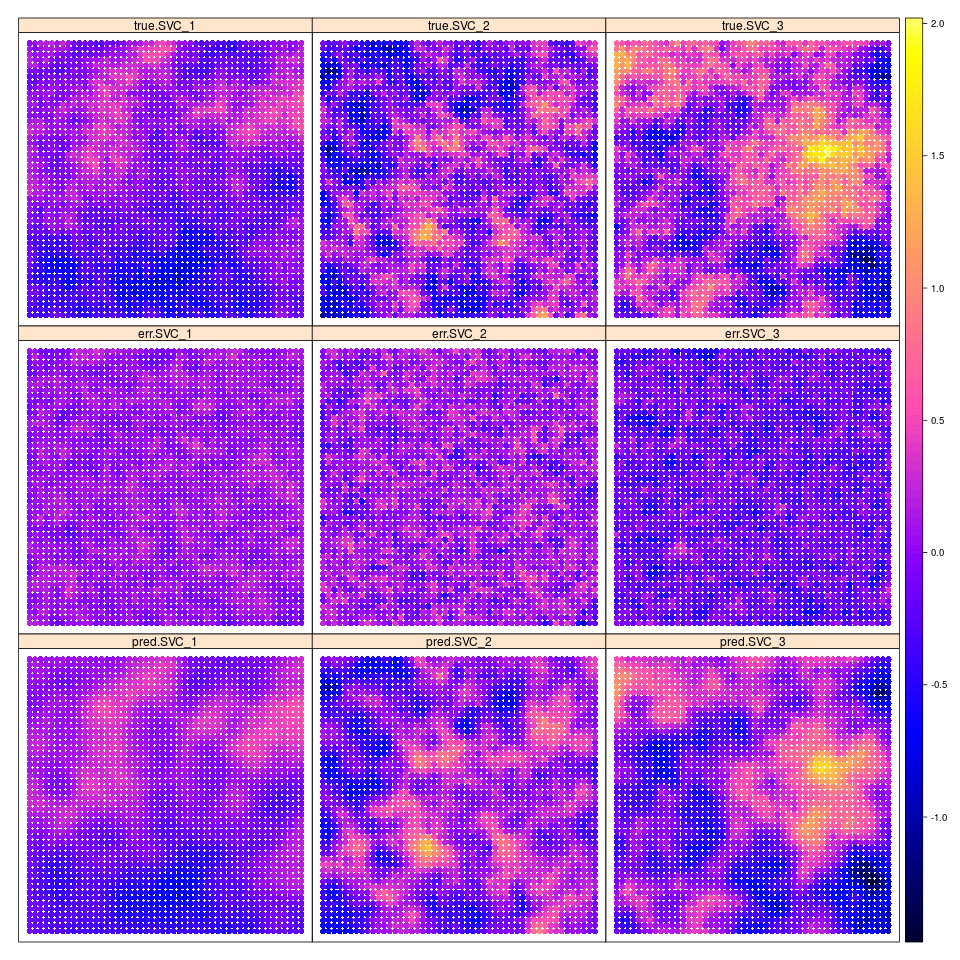We do not run the code since it takes a couple hours to do the MLE without parallelization, but here is the code to reproduce the figure:

# new m
m <- 50

# new SVC model
sp.SVC <- fullSVC_reggrid(m = m, p = p,
cov_pars = pars,
nugget = nugget.var)

spplot(sp.SVC, colorkey = TRUE)

# total number of observations
n <- m^2
X <- matrix(c(rep(1, n), rnorm((p-1)*n)), ncol = p)
y <- apply(X * as.matrix(sp.SVC@data[, 1:p]), 1, sum) + sp.SVC@data[, p+1]

fit <- SVC_mle(y = y, X = X, locs = coordinates(sp.SVC))

sp2500 <- predict(fit)

colnames(sp2500)[1:p] <- paste0("pred.",colnames(sp2500)[1:p])
coordinates(sp2500) <- ~loc_x+loc_y
all.SVC <- cbind(sp2500, sp.SVC[, 1:3])

# compute errors
all.SVC$err.SVC_1 <- all.SVC$pred.SVC_1 - all.SVC$SVC_1 all.SVC$err.SVC_2 <- all.SVC$pred.SVC_2 - all.SVC$SVC_2
all.SVC$err.SVC_3 <- all.SVC$pred.SVC_3 - all.SVC\$SVC_3

colnames(all.SVC@data) <- paste0(rep(c("pred.", "true.", "err."), each = p), "SVC_", rep(1:p, 3))

png(filename = "figures/SVCs_result_n2500_p3.png", width = 960, height = 960)
spplot(all.SVC[, paste0(rep(c("true.", "err.", "pred."), each = p),
"SVC_", 1:p)], colorkey = TRUE,
as.table = TRUE, layout = c(3, 3))
dev.off()